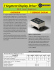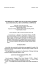Lesson 12: Dividing Segments Proportionately

Transcription

Lesson 12: Dividing Segments Proportionately
GEOMETR
Lesson 12
NYS COMMON CORE MATHEMATSCS CURRICULUM
Period:
Date
Lesson 12: Dividing Segments Proportionately
Learning Target
•
I can find midpoints of segments and points that divide segments two or more proportional or equal parts.
Opening Exercise (15 minutes)
Points/I(-4,5), S(12,13) and C(12,9) are plotted
on the coordinate grid
•
What is the length of ~AC1
1(0
•
What is the length of BC?
I
1
2
1
4
S
I
T
t
*
II
11 II
II
Mark the halfway point on AC and label it point .
What are the coordinates of pointP?
Mark the halfway point on BC and label it point
R. What are the coordinates of point R~>
Draw a segment from P to AB perpendicular to AC.
Mark the intersection point M. What are the
coordinates of M?
Draw a segment from R to >15 perpendicular to
BC. Mark the intersection point M. What are th<
coordinates of M?
Point M is called the
of AB.
Look at the coordinates of the endpoints and the midpoint. Can you describe how to find the coordinates of
the midpoint knowing the endpoints algebraically?
The general formula for the midpoint of a segment with endpoints
i,yi) and (x2,y2) using the average formula:
M (——-,——-J
(*,.»,)
(x2,y,)
GEOMETR
Lesson 12
NYS COMMON CORE MATHEMATICS CURRICULUM
Period:
Name
Date
Example 1. Find the midpoint of ST given 5(-2,8) and T(10, -4). (Sketch the situation)
K
"<•
T
M\('
)
/ —
Example 2. Find the point that is one-quarter of the way along of^ST given
7(10, -4). (Sketch the situation)
10
Example 3. Af (— 2, 10) is the midpoint of segment AB. If A has coordinates (4, —5), what are the
coordinates of B2~
~v ~T~O ~~
y-
*-ir
y
Example 4 . Given PQ and point R that lies on PQ such that point R lies - of the length of PQ from point P
along PQ.
-?
Use the given information to determine the following ratios:
PR-.PQ =
'
RQ:PQ=
2^/Q
PR-.RQ =
RQ:PR =
GEOMETR
NYS COMMON CORE MATHEMATICS CURRICULUM
LSSSOH 12
Period:
Name
Date
Examples. Given points A (—3,5) and 5(12,15), find the coordinates of the point, C, that sits -of the -way
along the segment AS, closer to A than it is to B. (Sketch the situation)
Y
10
>/
Divide the segment based on a part:whole ratio
To partition (divide) the segment into smaller parts when part to whole ratio is given:
1. Multiple the difference in x-coordinates (x2 — *i) and the difference in y-coordi nates (y2 — yj.) by
/ part .
whole
the given ratio ( - ).
Then add those products to the original point (*i,yi) to find the partition point (xp,yp).
Another way to look at it: Recall point-slope form of a linear equation, y — y± = m(x — x-^). By adding
to both sides, you get = m(x - xj + y^ . Finding a partition point uses a similar formula:
and
Division of the segment given as a part: part ratio -» convert to part:whole
Example 6. Find the point on the directed segment from (—3,0) to (5,8) that divides it in the ratio of 1: 3.
(Sketch the situation)
Example 7. Find the point on the directed segment from (-4,5) to (12,13) that divides it into a ratio of 1:7.
(Sketch the situation)
\ / \X
D&/T
v » i I'") -*^~^ » ~%Z i ~\—
U
\i~
l>
A >
N * *"
^-
'
*v
^/¥
GEOMETR
NYS COMMON CORE MATHEMATICS CURRICULUM
Lesson 12
Name
Period:
Date
CB
1
Example 8. Given points ,4(3, —9) and £(19, — 1), find the coordinates of point C such that — = -.
AC
9^
(Sketch the situation)
*-
-7
-? 7
-7
Example 9. Find the coordinates of point P along the directed line
3
segment AB so that the ratio of AP to PB is -.
{h'T~
J,
1,1ft-
_:
•I
V
?
o
CB
3
Example 10. Given points 4(3, -5) and fl(19, -1), find the coordinates of point C such that — = -.
4C
etch the situation
V
£
7
^

Geometry Worksheet Name: Hodge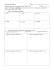Geometry Secondary Education MAFS.912.G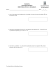Reteach Sec 1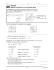The Midpoint Formula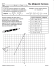STUDY GUIDE for Unit 1 Test Geometry 1. Z?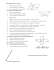WinGap Sketch Conversion Presentation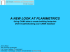HSM12CC_GM_06_08_CM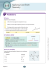TTCSI Services Talk final proposal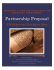Triple Integrals for Volumes of Some Classic Shapes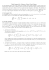Thomas Buckner Tom Hamilton jump the jump the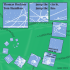5.1 Midsegment Theorem and Coordinate Proof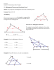o - ANMI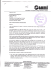C1 Worksheet D - WordPress.com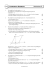Step ~1.5 hours on 22 CPUs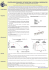Marketing Technology Strategy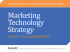Integrated PEPFAR-Supported Site List (iPSL)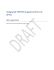TLC 3D SURVEY CAPTURING SOFTWARE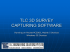The Tenuous Segment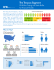Perceptual Color Space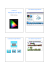Oregon 99W is the main highway through Newberg and Dundee. It